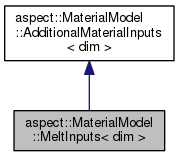ASPECT
aspect::MaterialModel::MeltInputs< dim > Class Template Reference
Inheritance diagram for aspect::MaterialModel::MeltInputs< dim >:[legend]

## Public Member Functions

MeltInputs (const unsigned int n_points)

void fill (const LinearAlgebra::BlockVector &solution, const FEValuesBase< dim > &fe_values, const Introspection< dim > &introspection) overridePublic Member Functions inherited from aspect::MaterialModel::AdditionalMaterialInputs< dim >

## Public Attributes

std::vector< double > compaction_pressures

std::vector< Tensor< 1, dim > > fluid_velocities

## Detailed Description

### template<int dim> class aspect::MaterialModel::MeltInputs< dim >

The MeltInputs provide the compaction pressures and melt (fluid) velocities, so that they can be used as additional inputs in heating or material models.

Definition at line 43 of file melt.h.

## § MeltInputs()

template<int dim>
 aspect::MaterialModel::MeltInputs< dim >::MeltInputs ( const unsigned int n_points )

Constructor. When the MeltInputs are created, all properties are initialized with signalingNaNs. This means that individual heating or material models can all attach the plugins they need, and in a later step they will all be filled together (using the fill function).

## § fill()

template<int dim>
 void aspect::MaterialModel::MeltInputs< dim >::fill ( const LinearAlgebra::BlockVector & solution, const FEValuesBase< dim > & fe_values, const Introspection< dim > & introspection )
overridevirtual

Fill the compaction pressures and fluid velocities.

## § compaction_pressures

template<int dim>
 std::vector aspect::MaterialModel::MeltInputs< dim >::compaction_pressures

Compaction pressure values $$p_c$$ at the given positions.

Definition at line 59 of file melt.h.

## § fluid_velocities

template<int dim>
 std::vector > aspect::MaterialModel::MeltInputs< dim >::fluid_velocities

An approximation for the fluid (melt) velocities at the given positions.

Definition at line 65 of file melt.h.

The documentation for this class was generated from the following file:
• /home/bob/source/include/aspect/melt.h